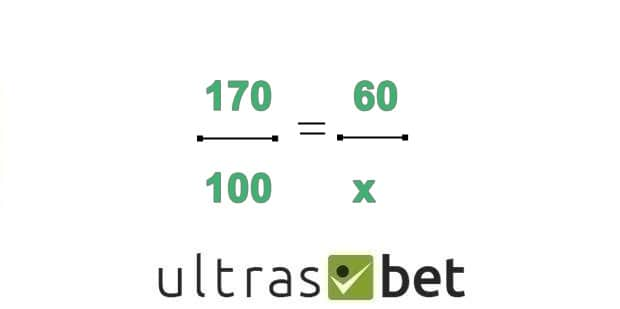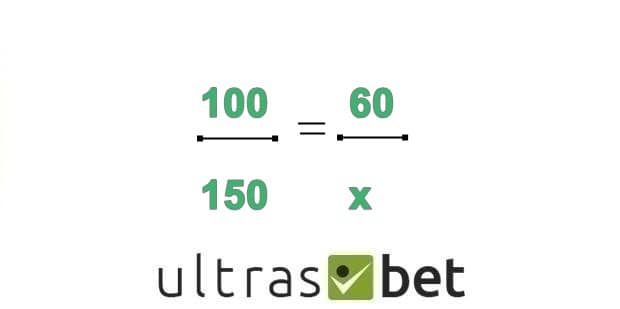First of all, we would like to start with explaining what are American, Decimal and Fractional odds. What they have in common is that they show the probability of a certain event to happen. You can also understand by them how much money you’re going to win, depending on your stake, in case the bet is winning.

When you see “+” or “” in front of a certain number such as “+250” or “-170”, it means that we are dealing with American betting odds. The “” and the “+” indicate whether you are betting on the favorite or on the underdog. Negative numbers show that you are betting on the favorite and the number after the “” symbol signifies the amount you need to bet, in order to win 100 USD. If the number is positive, it means that you are betting on the underdog and again, the number refers to the amount of money you’ll win if you bet 100 USD.

## How to Read Sports Betting Odds

For example, you have a game between the New England Patriots and the Kansas City Chiefs and the Patriots are favorite to win it with -170 while the Chiefs are the underdogs with +150. If you bet 60 USD on New England, you can use this formula, in order to calculate how much you are going to win:• Cross-multiply to get 170x = 6000, and then solve for “x
x= 6000/170
x = 35.29

Hence, if you wager 60\$ on the Patriots, you will win 95.29 USD which means that you will have a 35.29 USD profit.

However, if you bet 60\$ on Kansas City, you will have to use the following equation:• Cross-multiply to get 150x = 9000, and then solve for “x”.
x = 9000/150
x = 60

By wagering 60 USD on the Kansas City Chiefs, you will win 150.00 USD with a 90.00 USD profit.

## How to Read Odds in Sports

Calculating the implied probability with American odds will give you what is the percentage of a certain event to happen. Implied probability is always useful because you can check what the bookies are thinking and if it is different from what you are thinking, you can adjust your stake accordingly. For example, if you think that a team has a chance 70% chance to win a game but the bookies say that it has a 53% chance, it would be smart to bet on this team and receive a better payout than you thought.

For example, if we use Dallas Cowboys some game +110’ odds, we will use the following formula to calculate the probability of them to win the game:

In this case, we have odds of 110. We divide 100 by 110+100 which makes 47.6% chance of winning the game.

The same is applied for the negative odds, however, a different formula is being used:

The minuses here shouldn’t bother you. They are in the equation just to flip the sign of the negative US odds. So we have to divide -(-170) by -(-170)+100 which makes 63%.

The Decimal odds are the easiest to be understood, at least, based on my experience. The decimal odds are displayed like this: 2.40, 1.36, 1.88 and etc.

In order to calculate your winnings, you need to multiply your stake and odds. For example, if you decide to bet 60 USD on a market with 2.00 odds, you will win 120 USD with a 60 USD profit. You can also calculate the implied probability with decimal odds using the following equation:

And lastly, we will explain how the Fractional odds work. They are mainly used in the UK and they have its origins from the horse racings. They look like this: 9/5, 5/12, 1/3 and etc. The number on the left suggests how many times the outcome could fail while the number on the right tells us how many times the outcome would succeed.

For example, we have a game between the Los Angeles Lakers and the New York Knicks. The Lakers have odds of 8/13 which means that if this game takes place 21 times, the Lakers will lose just 8 of those 21 matchups. To calculate the implied probability, regarding this market, we take the number of times they are expected to win (13) and divide it by the total number of games (21). This results in a 61.9% chance of winning that particular game.

If you want to bet 10 USD on the Lakers to win the game, you can calculate your winnings by multiplying your wager by the quotient 8:13 (0.615). This means that if you win your bet of 10 USD, you will receive 6.15 USD as a profit and 16.15 USD in total.